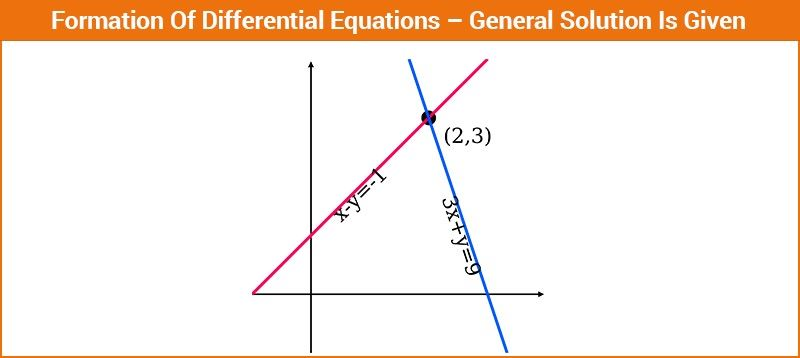# Formation of Differential Equations

For any given differential equation, the solution is of the form f(x,y,c1,c2, …….,cn) = 0 where x and y are the variables and c1 , c2 ……. cn are the arbitrary constants. To learn the formation of differential equations in a detailed way, you are provided with suitable differential equations examples below with few important steps. Differential equation formulas are important and help in solving the problems easily.To obtain the differential equation from this equation we follow the following steps:-

Step 1: Differentiate the given function w.r.t to the independent variable present in the equation.

Step 2: Keep differentiating times in such a way that (n+1) equations are obtained.

Step 3: Using the (n+1) equations obtained, eliminate the constants (c1 , c2 … …. cn).

## The solution of Differential Equations

The general solution of the differential equation is the relation between the variables x and y which is obtained after removing the derivatives (i.e., integration) where the relation contains arbitrary constant to denote the order of an equation. The solution of the first-order differential equations contains one arbitrary constant whereas the second-order differential equation contains two arbitrary constants. If particular values are given to the arbitrary constant, the general solution of the differential equations is obtained. To solve the first-order differential equation of first degree, some standard forms are available to get the general solution. They are:

• Variable separable method
• Reducible into the variable separable method
•  Homogeneous differential equations
• Non-homogeneous differential equations
• Linear differential equation
• Reducible into a linear differential equation
• Exact differential equations
• Linear differential equations with constant coefficients

Let us see a few differential equations examples to understand this concept clearly.

### Differential Equations Examples

 Let’s Work Out: Example 1: Find the differential equation corresponding to the equation $$\begin{array}{l} y = ae^x + be^{2x} + ce^{-3x} \end{array}$$ where a, b, c are arbitrary constants. Solution: Given: y = aex + be2x + ce-3x   y’ = aex + 2be2x – 3 ce-3x  y” = aex + 4be2x + 9 ce-3x  y’’’ = aex + 8be2x – 27 ce-3x  y’ – y = be2x – 4ce-3x——————– (1)  y” – y’ = 2be2x + 12ce-3x——————– (2) y”’ – y” = 4be2x – 36ce-3x——————– (3) Now,with the help of matrices and by using elimination technique for eliminating b and c we get, $$\begin{array}{l}\begin{bmatrix} y’-y & 1 & -4 \\ y”-y’ & 2 & 12\\ y”’-y” & 4 & -36 \end{bmatrix} = 0\end{array}$$ This matrix can be simplified as, $$\begin{array}{l}\begin{bmatrix} y’-y & 1 & -1 \\ y”-y’ & 2 & 3\\ y”’-y” & 4 & -9 \end{bmatrix} = 0\end{array}$$ Expanding we get (y’-y)(-18-12) -1 (-9(y”-y’) – 3(y”’ – y”)) – 1(4(y” – y’) – 2(y”’ – y”)) = 0  -30(y’-y) + 5(y” – y’) + 5(y”’ – y”) = 0   (y”’ – y”) + (y” – y’) – 6(y’ – y) = 0   y”’ – 7y’ + 6y = 0   d3y/dx3 – 7  dy/dx + 6y = 0  This is the required differential equation. Example 2: Find the differential equation of all the hyperbolas whose axes are along both the axes. Solution: The standard equation of a hyperbola is x2/a2 – y2/b2= 1, whose transverse and conjugate axes are along the coordinate axes. As there are two arbitrary constants, to eliminate them we need to differentiate the standard equation of hyperbola twice. Differentiating the above equation with respect to x, we get, 2x/a2 – 2yy’/b2 = 0….(1) 2x/a2 = 2yy’/b2  b2/a2 = yy’/x…..(2) Differentiating (1) w. r. t. x again, we get, 2/a2 – 2/b2 ((y’)2 + yy”) = 0 2/a2 = 2/b2 ((y’)2 + yy”) b2/a2 =y’2 + yy” …….. (3) Equating the values of b2/a2 from (2) and (3) yy’/x = y’2 + yy”  Or,  y (dy/dx) = x(dy/dx)2 + xy (d2y/dx2) This represents a differential equation of second order obtained by eliminating two parameters. Example 3: Find the differential equation of the family of circles of radius 5cm and their centres lying on the x-axis. Solution: Let the centre of the circle on x-axis be (a,0). The equation of such a circle can be given as: (x-a)2 + y2 = 52  ……(1) (x-a)2 + y2 = 25  Differentiating this equation with respect to x, we get, 2(x-a) + 2y dy/dx = 0 Taking 2 as common and eliminating, (x-a) = -y (dy/dx) Substituting the value of (x – a) in equation (1), we get y2(dy/dx)2 + y2 = 25 This is the required differential equation.

Thus, you have seen how the differential equations are formed. Using this concept one can form various kinds of differential equations. Download BYJU’S -The Learning App to make this journey of differential equations easier.